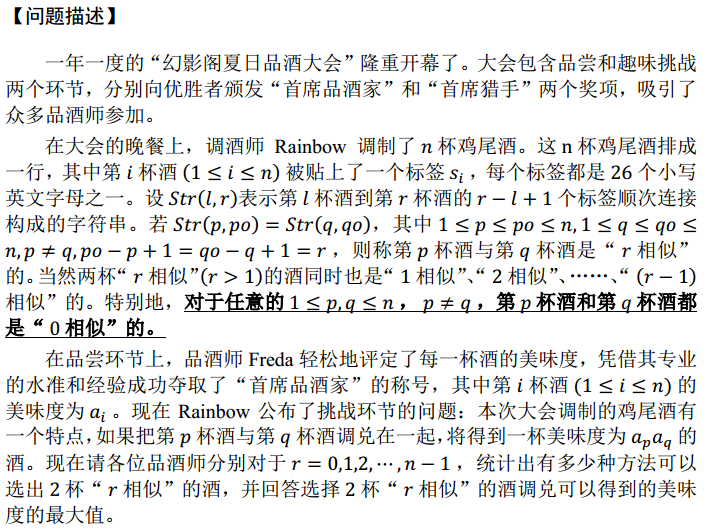「NOI2015」品酒大会 - 后缀自动机 | Bill Yang's Blog

## 题目大意## 题目分析

$cnt[\left|max(now)\right|]+=C_{end\text{-}pos(now)}^2$

$ans[\left|max(now)\right|]=\max\lbrace ans[\left|max(now)\right|],end$-$max[now]\times end$-$max[now],end$-$min[now]\times end$-$min[now])\rbrace$

0%